# The quadratic equation, the theorem of vieta

Definition: quadratic equation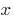— an equation of the formwhere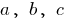is some number, and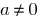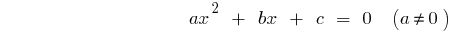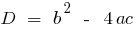the discriminants of the quadratic equation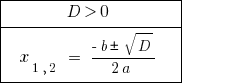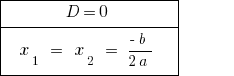If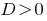the equation has two distinct roots.

Ifthe equation has two equal roots. When counting the number rozwaski is considered one value of the root.

When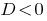— equation roots there.

### Master equation (a = 1)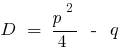the discriminants of the combined equation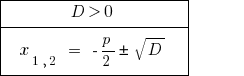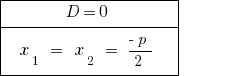At— master equation has two distinct roots.

When— the equation has two equal roots. When counting the number rozwaski is considered one value of the root.

If— the equations do not have roots.

## Vieta theorem in the General case

If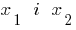the roots of the quadratic equation, then## The vieta theorem for the master equation (a=1)

Ifthe consolidated roots of the quadratic equation, then### The inverse theorem the theorem of vieta

Theorem: If the sum of the two numbersequal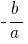, and the product still, these numbers are roots of the quadratic equation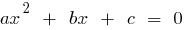.

Theorem (master equation): If the sum of the two numbersequal, and the product still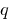, these numbers are roots of the quadratic equation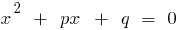.

## Decomposition of a square trinomial into factors

Ifthe roots of the quadratic trinomial equal to zero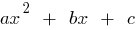(i.e. the roots of the equation)thenIf the discriminants square trinomial is equal to zero (),and then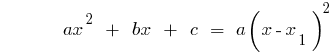### Example. Decomposition of the trinomial.

1.when2.3.when4.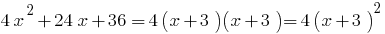Tags:
Chapter:
Versions in other languages:
Share with friends: